# Language Model Note (SLP Ch03)

## Ngram

• Count up the frequency and divide (use maximum likelihood estimation or MLE):

• Example: $P(you | i\ love) := \frac {c(i\ love\ you)}{c(i\ love)}$
• More Generally: $P(w_1^n) = P(w_1)P(w_2 | w_1)P(w_3|w_1^2)...P(w_n|w_1^{n-1}) = \prod_{i=1}^{n} P(w_i|w_1^{i-1})$
• For N-gram

• Next Word: $P(w_n|w_1^{n-1}) \approx P(w_n|w_{n-N+1}^{n-1}) = \frac {C(w_{n-N+1}^{n-1}w_n)}{C(w_{n-N+1}^{n-1})}$
• prob of sentence: $P(w_1^n) \approx \prod_{i=1}^{n} P(w_i|w_{i-1}...w_{i-N+1})$
• For Bigram
• prob of sentence: $P(w_1^n) \approx \prod_{i=1}^{n} P(w_i|w_{i-1})$
• Next Word: $P(w_n|w_{n-1}) = \frac {C(w_{n-1}w_n)}{\sum_w C(w_{n-1} w)} = \frac {C(w_{n-1}w_n)}{C(w_{n-1})}$

## Why Bi-gram or Tri-gram

While this method of estimating probabilities directly from counts works ﬁne in many cases, it turns out that even the web isn’t big enough to give us good estimates in most cases. This is because language is creative; new sentences are created all the time, and we won’t always be able to count entire sentences. Even simple extensions of the example sentence may have counts of zero on the web (such as “Walden Pond’s water is so transparent that the”).

Similarly, if we wanted to know the joint probability of an entire sequence of words like its water is so transparent, we could do it by asking “out of all possible sequences of ﬁve words, how many of them are its water is so transparent?” We would have to get the count of its water is so transparent and divide by the sum of the counts of all possible ﬁve word sequences. That seems rather a lot to estimate!

• 语言具有极强的创造性（尤其是中文！！），它是无穷的，我们无法数完所有的句子；而且，也正是如此，会导致很多并不复杂的句子出现次数为 0，比如：“我喜欢张馨予”，这句话可能在语料中压根就不存在，那模型就会认为这句话的概率为 0，但这句话其实是很普通的一句话。

• 另一方面，要数出 “我喜欢张馨予” 的次数，我们需要把语料全部变成六元短语。比如我们假设语料是这么一句话：“我喜欢吃面，我不喜欢张雨绮，我喜欢张馨予。”因为我们事先并不知道 “我喜欢张馨予” 不存在，所以只好把语料变成：“我喜欢吃面，|喜欢吃面，我|欢吃面，我不|……“，然后去数。但是这个规模就太大了（因为 N 越大，不重复的越多）

Trigram 的计算过程：

• 数据规模不大时：collections.Counter()
• 数据规模太大时：先把语料全部处理成 Ngram，用空格或其他分隔符分开存储好；逐行读入，逐词存入字典，如果字典中已有则 +1，否则为 1。提示：所有读取操作用 generator，这种操作方式基本不耗内存，而且可以处理任意大的语料。

## Smoothing

• 处理 OOV（未登录词）
• 选择固定词表，没有在词表中的词为 UNK
• 选择固定数量的词或频次高于某个数的词，其余词为 UNK
• Why Smoothing
• words that are in our vocabulary (they are not unknown words) but appear in a test set in an unseen context (for example they appear after a word they never appeared after in training).
• 即词在词表中，但测试/预测数据上部分词的组合训练数据中没出现过，这时候就需要 Smoothing。比如上面的例子，“张、馨、予” 三个字在词表中，如果训练数据上没有 “张馨予”，测试数据上出现了，我们不能说 “张馨予” 这个词出现的概率为 0。

### Laplace Smoothing

• Laplace smoothing merely adds one to each count (hence its alternate name addone smoothing). Since there are V words in the vocabulary and each one was incremented, we also need to adjust the denominator to take into account the extra V observations.
• What happens to our P values if we don’t increase the denominator? The P value will bigger than 1
• Instead of changing both the numerator and denominator, it is convenient to describe how a smoothing algorithm affects the numerator, by deﬁning an adjusted count c ∗ .
• Bigram:
• 这个很简单，也很直观，如果你要给 $C(w_{n-1}w_n)$ 加 1，意味着 $C(w_{n-1})$ 要加 1，那所有词就是要加 V（词表大小）

• Although add-k is useful for some tasks (including text classiﬁcation), it turns out that it still doesn’t work well for language modeling, generating counts with poor variances and often inappropriate discounts (Gale and Church, 1994).

### Backoff and Interpolation

• Backoff: we only “back off” to a lower-order N-gram if we have zero evidence for a higher-order N-gram.
• Interpolation: we always mix the probability estimates from all the N-gram estimators, weighing and combining the trigram, bigram, and unigram counts.
• How are these λ values set?
• learned from a held-out corpus
• A held-out corpus is an additional training corpus that we use to set hyperparameters like these λ values, by choosing the λ values that maximize the likelihood of the held-out corpus.
• One way: EM
• discount
• In order for a backoff model to give a correct probability distribution, we have to discount the higher-order N-grams to save some probability mass for the lower order N-grams.
• 如果高阶的 Ngram 不存在，用低阶替换时需要 discount，否则会导致低阶的系数暴增。
• Just as with add-one smoothing, if the higher-order N-grams aren’t discounted and we just used the undiscounted MLE probability, then as soon as we replaced an N-gram which has zero probability with a lower-order N-gram, we would be adding probability mass, and the total probability assigned to all possible strings by the language model would be greater than 1! In addition to this explicit discount factor, we’ll need a function α to distribute this probability mass to the lower order N-grams.
• Katz backoff
•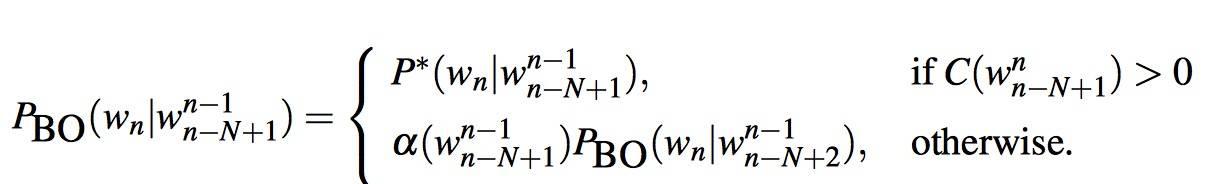• Katz backoff is often combined with a smoothing method called Good-Turing.

### Kneser-Ney Smoothing

• One of the most commonly used and best performing N-gram smoothing methods is the interpolated Kneser-Ney algorithm (Kneser and Ney 1995, Chen and Goodman 1998).

• Kneser-Ney has its roots in a method called absolute discounting. Discounting of the counts for frequent N-grams is necessary to save some probability mass for the smoothing algorithm to distribute to the unseen N-grams.

• 大规模语料上的统计 Bigram 结果，训练数据出现的次数与同等规模的 held-out 语料出现次数比为 4:3

• Absolute discounting formalizes this intuition by subtracting a ﬁxed (absolute) discount d(0.75) from each count.

•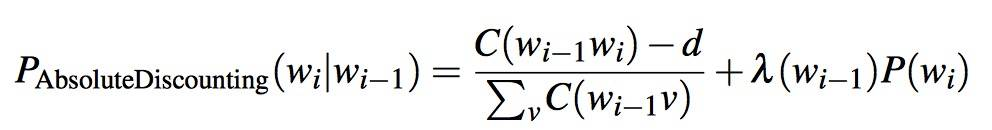• the second term the unigram with an interpolation weight λ.
• Kneser-Ney discounting (Kneser and Ney, 1995) augments absolute discounting with a more sophisticated way to handle the lower-order unigram distribution.

• P_CONTINUATION: We hypothesize that words that have appeared in more contexts in the past are more likely to appear in some new context as well. 如果一个词以前更容易与别的词组成词组，那么在新的文本中也会更容易组成词组。比如：“蝶” 出现的次数远高于 “榨”（因为 “蝴蝶” 出现的次数高），但 “蝶” 组合成词的可能性（基本上只有 “蝴蝶”）低于 “榨”，所以在给定上文的情况下，下一个字更可能是 “榨” 而不是 “蝶”。注：词频参考
• The Kneser-Ney intuition is to base our estimate of P CONTINUATION on the number of different contexts word w has appeared in, that is, the number of bigram types it completes. The number of times a word w appears as a novel continuation can be expressed as:
•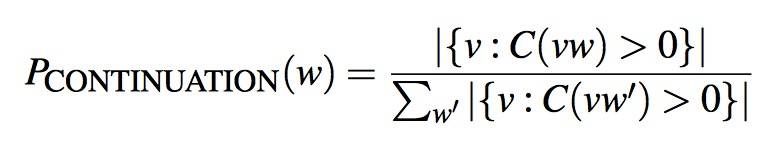• 其实就是给能组成更多 Bigram 的单个词更高的概率分布，A frequent word (“蝴”) occurring in only one context (“蝶”) will have a low continuation probability.
• The ﬁnal equation for Interpolated Kneser-Ney smoothing is

•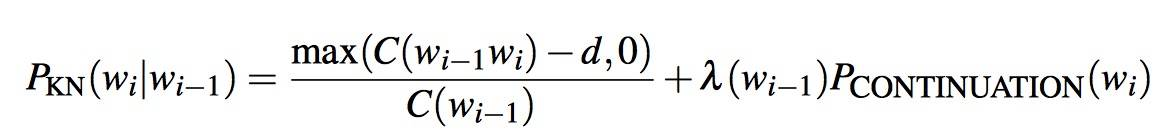• The λ is a normalizing constant that is used to distribute the probability mass we’ve discounted: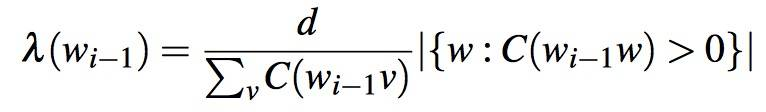• The ﬁrst term $\frac {d} {C(w_{i-1\ })}$ is the normalized discount.
• The second term $\lvert {w : C(w_{i−1}w) > 0} \rvert$ is the number of word types that can follow $w_{i−1}$ or, equivalently, the number of word types that we discounted; in other words, the number of times we applied the normalized discount.
• The general recursive formulation is as follows:
•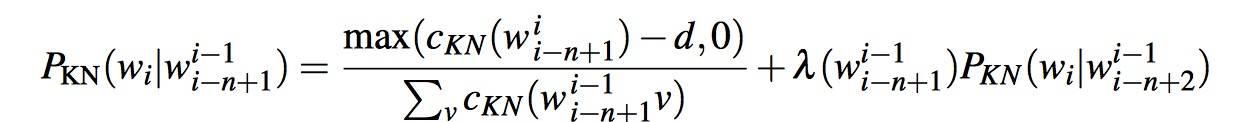•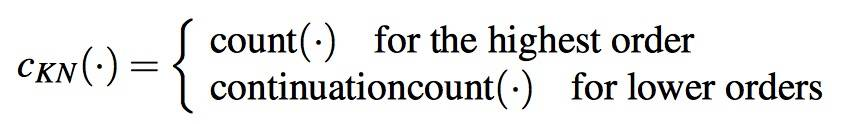•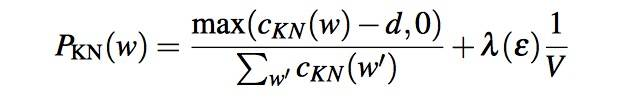The best-performing version of Kneser-Ney smoothing is called modiﬁed KneserNey smoothing, and is due to Chen and Goodman (1998). Rather than use a single ﬁxed discount d, modiﬁed Kneser-Ney uses three different discounts d1 , d2 , and d3+ for N-grams with counts of 1, 2 and three or more, respectively.

### The Web and Stupid Backoff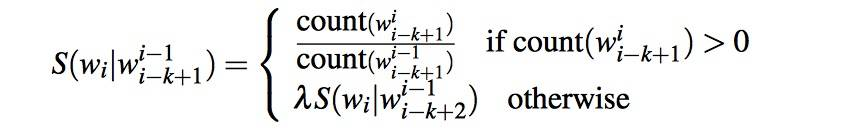S is score, not probability.
The backoff terminates in the unigram, which has probability S(w) = count(w)/N .
Brants et al. (2007) ﬁnd that a value of 0.4 worked well for λ.

k = 3，S（予|张馨）= C（张馨予）/C（张馨）or 0.4 * C（馨予）/C（馨）

## Evaluating Language Models

• The best way to evaluate the performance of a language model is to embed it in an application and measure how much the application improves. Such end-to-end evaluation is called extrinsic evaluation.
• An intrinsic evaluation metric is one that measures the quality of a model independent of any application. For an intrinsic evaluation of a language model we need a test set.
• Sometimes we use a particular test set so often that we implicitly tune to its characteristics. We call the initial test set the development test set or, devset.
• what does it mean to “model the test set”? The answer is simple: whichever model assigns a higher probability to the test set—meaning it more accurately predicts the test set—is a better model.
• In practice, we often just divide our data into 80% training, 10% development, and 10% test.

### Perplexity

• In practice we don’t use raw probability as our metric for evaluating language models, but a variant called perplexity.
• For a test set W = w1, w2 … wN:
• The higher the conditional probability of the word sequence, the lower the perplexity.
• What we generally use for word sequence in Eq above is the entire sequence of words in some test set.
• Since this sequence will cross many sentence boundaries, we need to include the begin- and end-sentence markers <s> and </s> in the probability computation.
• We also need to include the end-of-sentence marker </s> (but not the beginning-of-sentence marker <s>) in the total count of word tokens N.
• Any kind of knowledge of the test set can cause the perplexity to be artiﬁcially low. The perplexity of two language models is only comparable if they use the same vocabulary.

### Advanced: Perplexity’s Relation to Entropy

• By making some incorrect but convenient simplifying assumptions, we can compute the entropy of some stochastic process by taking a very long sample of the output and computing its average log probability.
• PPT 上的 A Refresher on Evaluation: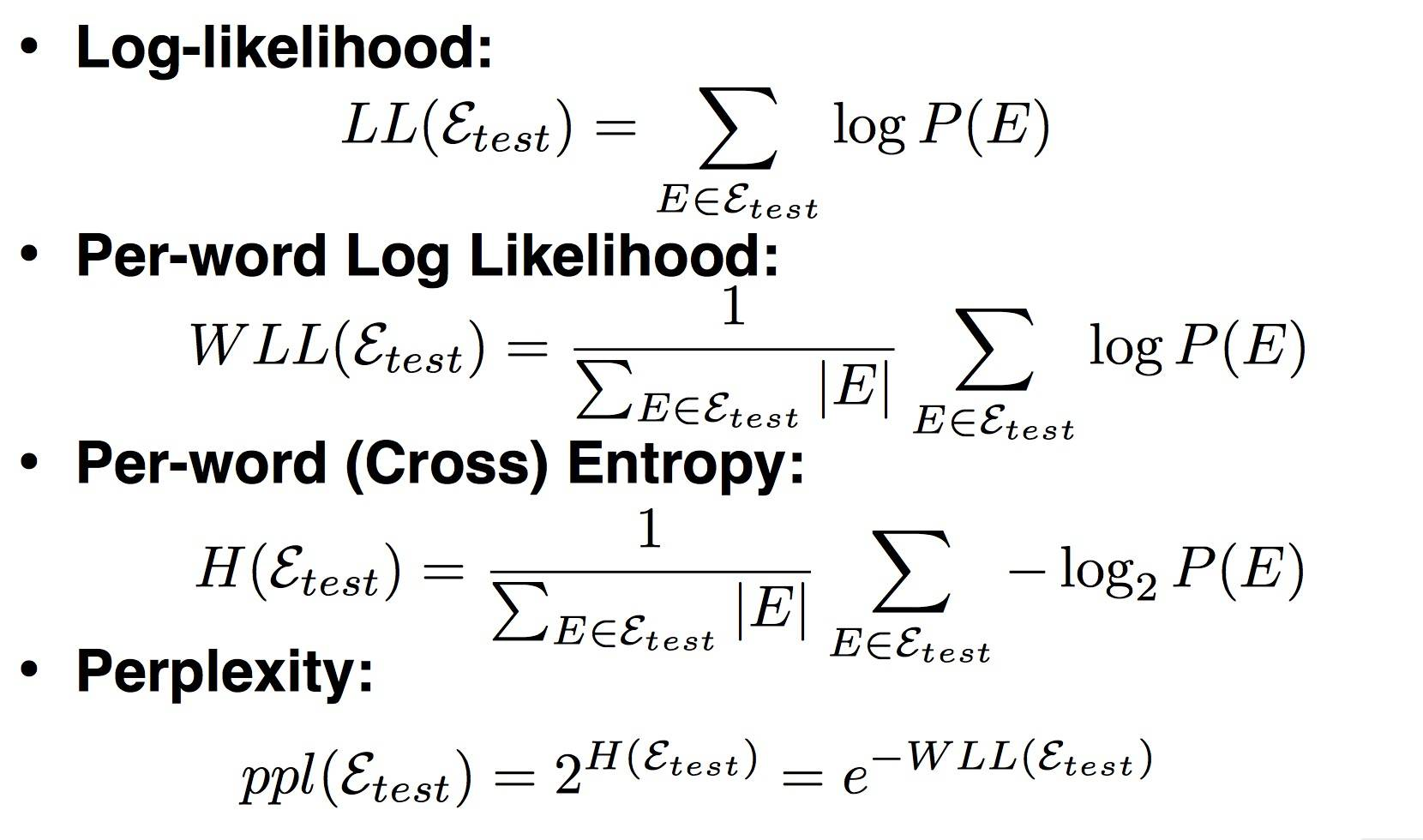## What Can we Do w/ LMs?

• Score sentences
• Generate sentences
• while didn’t choose end-of-sentence symbol
• calculate probability
• sample a new word from the probability distribution

## Problems and Solutions?

• Cannot share strength among similar words
• example: she _bought_ a _car_, she _purchased_ a _car_ || she _bought_ a _bicycle_, she _purchased_ a _bicycle_
• solution: class based language models
• Cannot condition on context with intervening words
• example: _Dr._ Jane _Smith_ || _Dr._ Gertrude _Smith_
• solution: skip-gram language models
• Cannot handle long-distance dependencies
• example: for _tennis_ class he wanted to buy his own _racquet_ || for _programming_ class he wanted to buy his own _computer_
• solution: cache, trigger, topic, syntactic models, etc.

## Summary

• Language models offer a way to assign a probability to a sentence or other sequence of words, and to predict a word from preceding words.
• n-grams are Markov models that estimate words from a fixed window of previous words. n-gram probabilities can be estimated by counting in a corpus and normalizing (the maximum likelihood estimate).
• n-gram language models are evaluated extrinsically in some task, or intrinsically using perplexity. The perplexity of a test set according to a language model is the geometric mean of the inverse test set probability computed by the model.
• Smoothing algorithms provide a more sophisticated way to estimate the probability of n-grams. Commonly used smoothing algorithms for n-grams rely on lower-order n-gram counts through backoff or interpolation. Both backoff and interpolation require discounting to create a probability distribution.
• Kneser-Ney smoothing makes use of the probability of a word being a novel continuation. The interpolated Kneser-Ney smoothing algorithm mixes a discounted probability with a lower-order continuation probability.